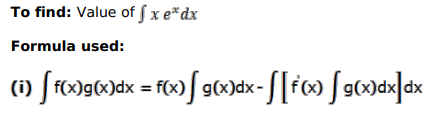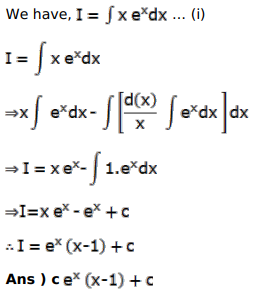# Mark against the correct answer in each of the following:

Question:

Mark $(\sqrt{)}$ against the correct answer in each of the following:

$\int x e^{x} d x=?$

A. $e^{x}(1-x)+C$

B. $e^{x}(x-1)+C$

C. $e^{x}(x-1)+C$

D. none of these

Solution: